# background, theory .pdf

### File information

Original filename: background, theory.pdf

This PDF 1.5 document has been generated by TeX / MiKTeX pdfTeX-1.40.12, and has been sent on pdf-archive.com on 30/05/2013 at 22:29, from IP address 142.157.x.x. The current document download page has been viewed 830 times.
File size: 133 KB (5 pages).
Privacy: public file

background, theory.pdf (PDF, 133 KB)

### Document preview

1

Background: Theory

We would like to search a set of models, M, in order to predict observations from an unknown datagenerating process q, when we only have a finite data set D of independent observations from q. A
principle hazard of model searches is losing predictive power by choosing a model that describes the
data better than the process that generated it. Our aim is to understand and avoid overfit in model
searches. Therefore, we will build handles on predictiveness, overfit and the information in D and
suggest an overfit preventing model search algorithm.
To clarify the discussion, observe that each parametrization of each predictive model induces a distribution over the event set, and that two distinct parametrized models inducing the same distribution
make the same predictions. Overfit is a funtion of the parameters only through the distribution on
the event set induced by the parametrized model. Therefore, we will use the term “model” for the
equivalence class of parametrized models inducing the same distribution. A search of parametric forms
followed by a parametrization will therefore be seen as a search across classes of models followed by
a model search in the chosen class.

1.1

The predictiveness of m on data from p

The ability of a model m to predict observations generated from a model p is its asymptotic ability
to count the observations drawn independently from p:
Definition 1. The predictiveness of model m on data from model p is the average asymptotic expected
likelihood of m given data from p, denoted:

i
h
1
n
L(m; p) ≡ lim E L(m; Xn )
n→∞ Xn ∼p

1

Where Xn is a random sample of n independent observations drawn from the distribution induced by
model p. The average is taken geometrically because the likelihood almost surely declines geometrically with linear increases in the sample size.
The following theorem and corollary are computationally and theoretically important:
Theorem 1. If p is a discrete probability vector with k categories, then:


lim

n→∞

n
np1 . . . npk

1/n
= lim

n→∞

!1/n

n!

=

Qk

i=1 (npi )!

k
Y

(
pi−pi = exp −

i=1

k
X

)
pi ln pi

(1)

i=1

Corollary 1. For discrete probability vectors m and p:

L(m; p) =

p
k 
Y
mi i
i=1

pi

(
= exp −

k
X
i=1

pi
pi ln
mi

)
(2)

= exp {−DKL (p; m)}

Where DKL (p; m) is the Kullback-Leibler divergence of p given m. Notice that the predictiveness of
m on p is 1 if and only if m = p, in the sense that they induce the same distribution, and that the
predictiveness is zero if and only if m assigns a zero probability to an event of nonzero probability
under p.

1.2

Overfit

Let e(D) denote the distribution that gives precisely the observed relative frequencies of events in D
as the probability of these events, and let us call this the empirical model. Then we can define the
amount of overfit in a model choice as the extent to which the chosen model predicts data from the
empirical model better than it predicts data from the generating process, q:
Definition 2. The amount of overfit in a model choice, m, is given by
2

V (m, D, q) = L(m; e(D)) − L(m; q)

The goal of avoiding overfit is to increase predictiveness by refraining from learning information from
the data, D, that doesn’t describe the data generating process. The amount of overfit for each model
m on D is a function of the data. Therefore, any model search that uses an a priori compensation
to avoid overfit does not use information in D that could be used to improve its predictiveness by
estimating the overfit as a function of D. If we are to use the notion of “simplicity” to think about
avoiding overfit, then we ought to use an a posteriori definition of simplicity. Notice that both choosing
a “simple enough” parametric form before fitting it to the data and including a punishment for model
complexity in a Bayesian prior before updating use a priori methods to avoid overfit.

1.3

Information in D

The logic of statistical hypothesis testing can be used to avoid rejecting model choices that might predict the data-generating process even when the current observations are relatively unlikely according
to the models: If a model m induces the same distribution over the event set as the data generating
process q, then the p-value of m given data D from q is uniformly distributed on the unit interval. If
the p-value of a model m on the data is less than some α, then either m does not induce the same
distribution as q, or the p-value was drawn to be less than α from a uniform distribution on the unit
interval. Using a smaller significance level α makes it less likely that you will reject model choice m
due to unpredictive information in D. So we propose the following definition:
Definition 3. The information in D at the α-level is:

Iα (D, M) ≡ {m ∈ M | P-value(D; m) &lt; α}
3

That is, the α-level information in D is defined as the set of models in M that are rejected by a
hypothesis test with significance level α. This definition places the information in D in the context of
a model search; learning nothing means not being able to rule out any additional models from your
search.

1.4

The α-level underfit score

Let us treat Aα ≡ M \ Iα (D, M) as the set of candidate models; the set of models that might
induce the same distribution over the event set as the data generating process. Then we can write
the following definition:
Definition 4. The predictiveness profile of model m in candidate set Aα is:

L(m; Aα ) ≡ {L(m; p) | p ∈ Aα }

Since we don’t necessarily have a measure on Aα , the most natural function of the predictiveness
profile to use as a score for a model search is the “worst case predictiveness”, which we will define as:
Definition 5. The α-level underfit score of m given candidate set Aα ⊂ M is:

U Fα (m, M) ≡ inf L(m; p)
p∈Aα

We call this the “α-level underfit score” because it assigns the worst predictiveness that is possible
for each model as its score, if it is given or assumed that there is a model in M which induces the
same distribution as the data generating process and that the α level we have chosen is “the right
significance level”. That is, it aims to give models the worst score that is might eventually achieve,

4

given the data. In principle, models with better worst case scenarios are more robust to overfitting
than models with more severe worst cases.

1.5

Aggression and Avoiding Overfit

In the context of a model search there is no reason, in principle, why we should prefer one significance
level over another. Lower significance levels read less information from the data (they rule out fewer
models) than higher ones. Each will imply different worst case predictivenesses for each model. Using
a very low significance level will give a very conservative α-level underfit scoring of models, while using
a high significance level will give a more aggressive choice. Therefore, we will define an “information
preferences” that give weight or importance to each significance level:
Definition 6. An aggression profile is a distribution on the levels of statistical significance, i.e. a
probability measure on the unit interval.
We can now integrate the α out of α-underfit scores using a given aggression profile as the integrating
measure, to suggest a scoring:
Definition 7. The underfit score of m relative to the aggression profile µ is:

Z

1

U Fα (m, M)dµ

U F (m; µ) =
0

This model search score compensates for overfit as a function of the data, or more specifically, it
compensates for overfit as a function of the information in the data at every significance level. The
performance of this model search method will be compared to many Bayesian choice rules for discrete
multinomial data, in the next section.

5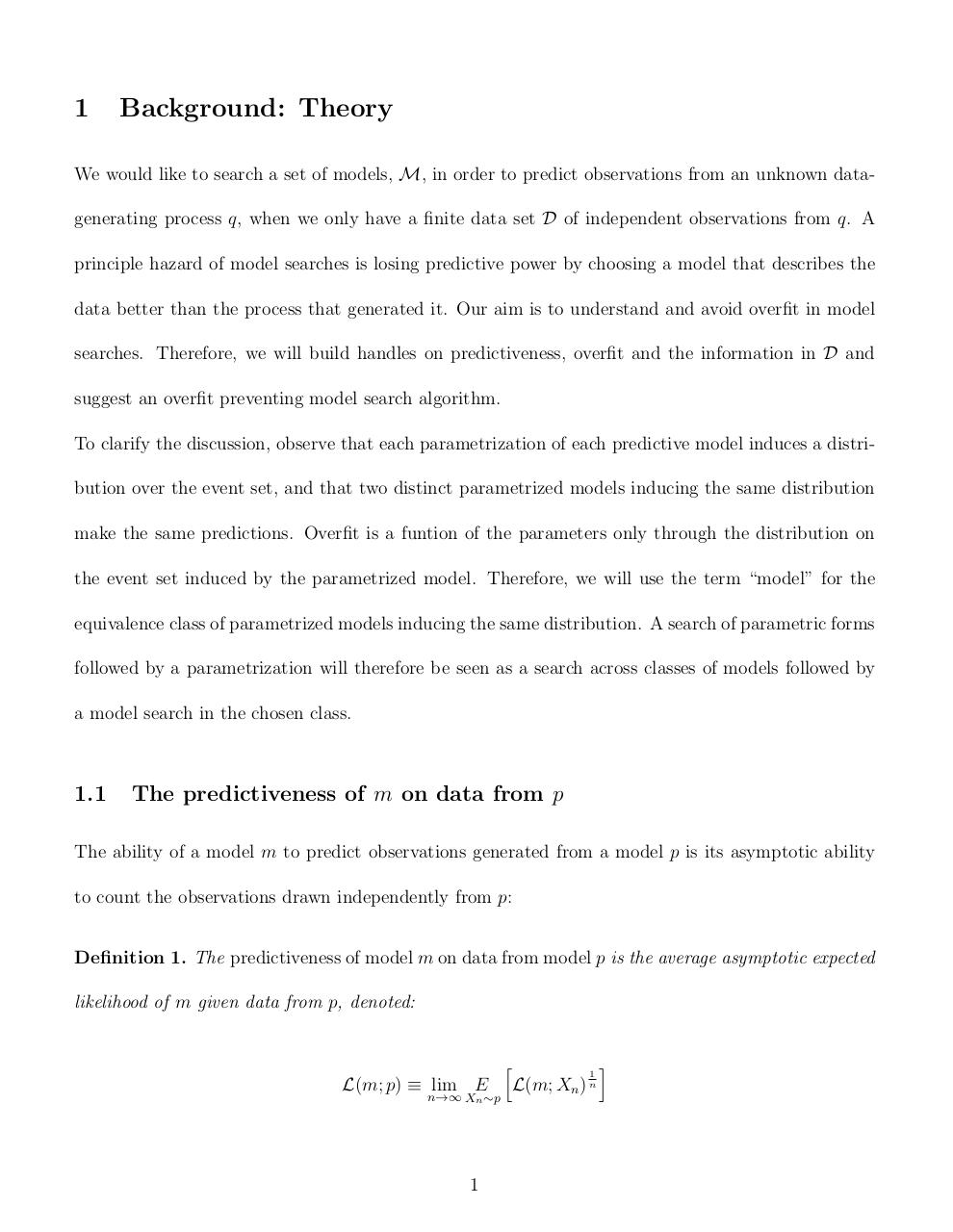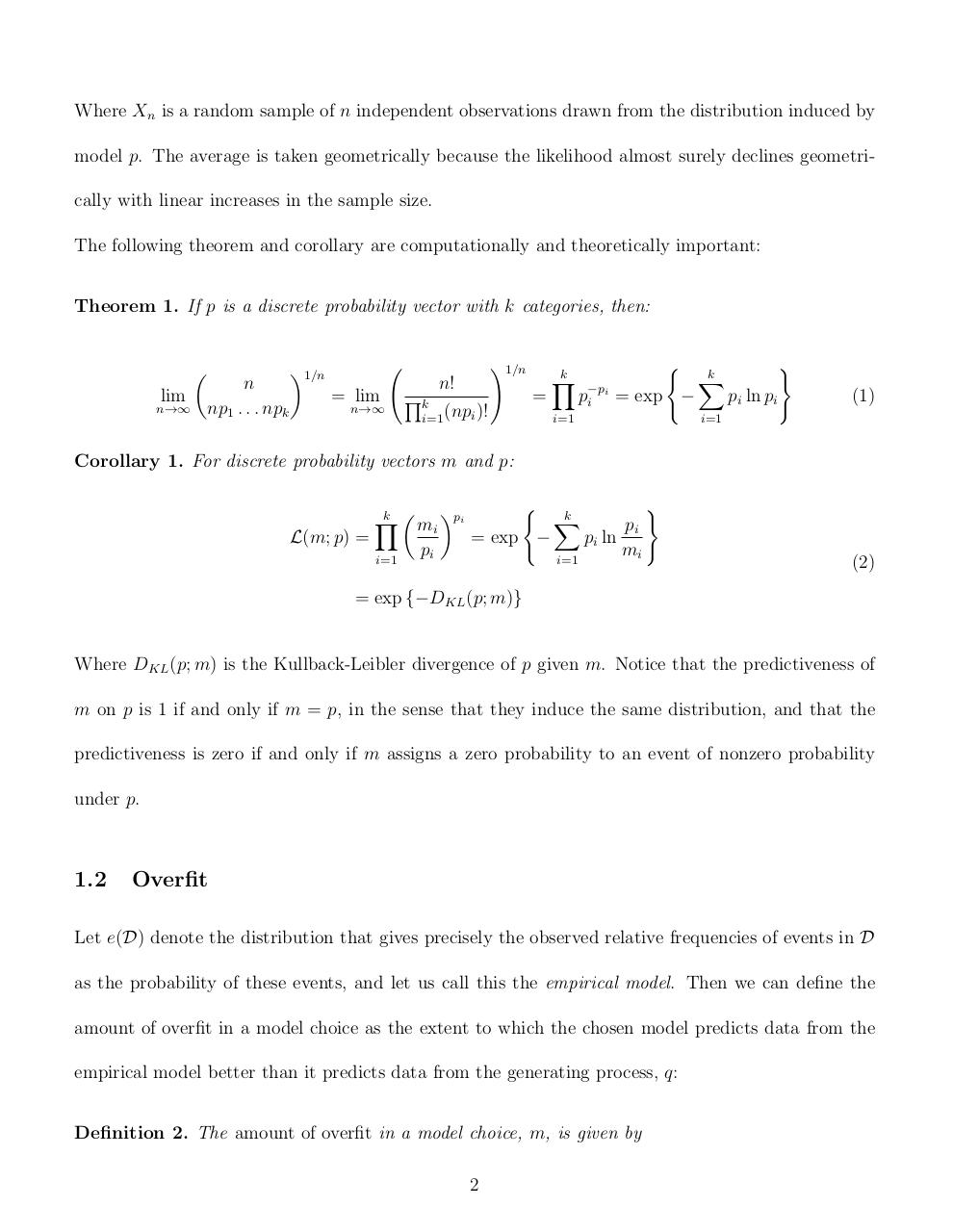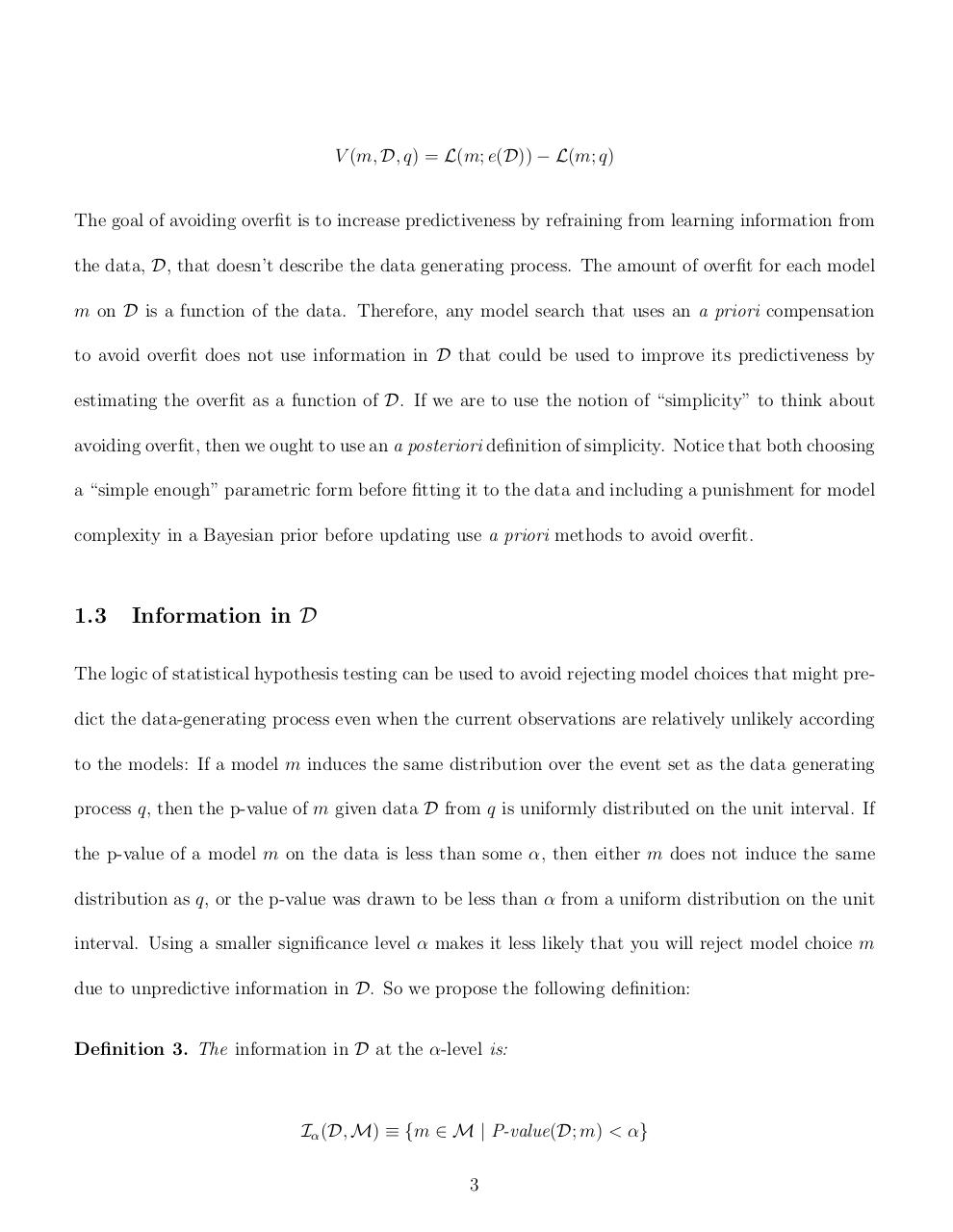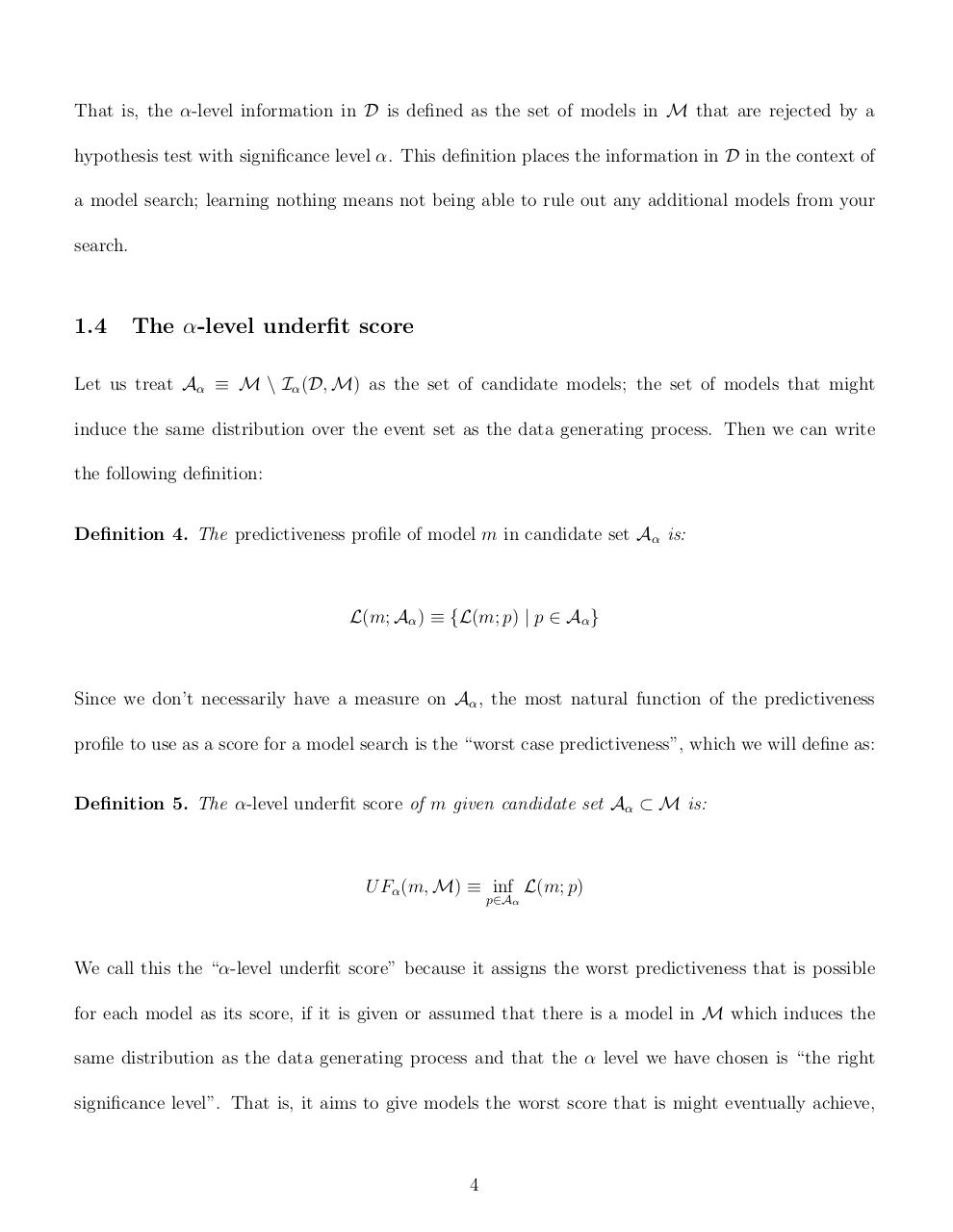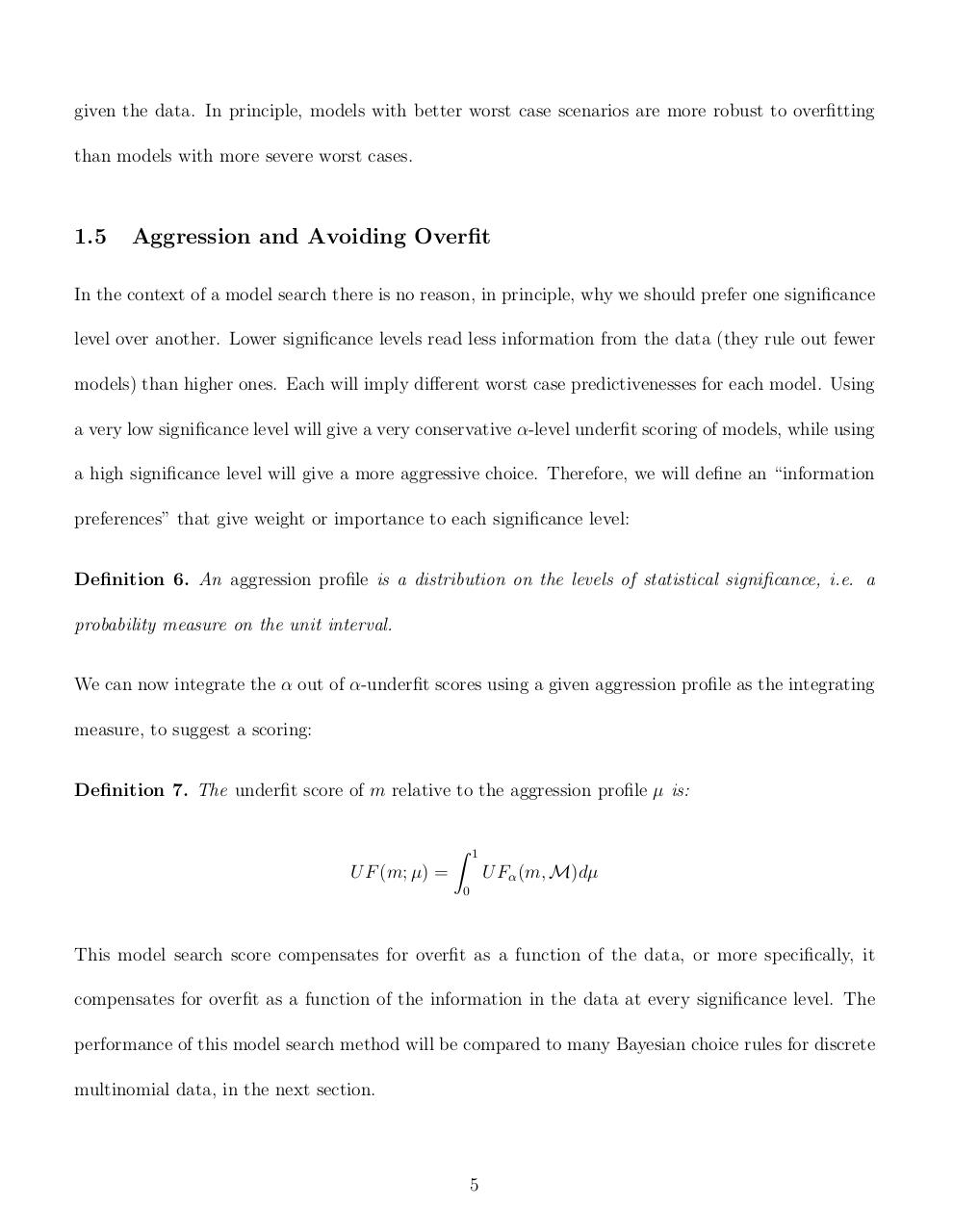#### HTML Code

Copy the following HTML code to share your document on a Website or Blog

#### QR Code### Related keywords